# Mesh data functions

Minitab includes example functions that are defined in the macro file USERFUNC.MAC, which is located in the Macros folder for your language. You can add your own custom functions to this file.

## Bivariate normal (function 1)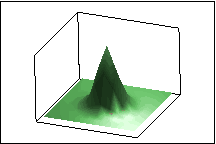Where:

### Parameters

You can specify values for the following parameters (in order):
• μ1
• σ1 (cannot equal 0)
• μ2
• σ2 (cannot equal 0)
• ρ (−1 < ρ < 1)

## Bowl (function 2)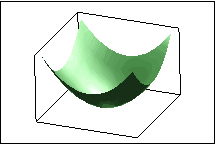### Parameters

You can specify values for the following parameters (in order):
• A (cannot equal 0)
• B (cannot equal 0)
• C (cannot equal 0)

## Cone (function 3)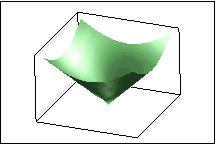### Parameters

You can specify values for the following parameters (in order):
• A (cannot equal 0)
• B (cannot equal 0)
• C (cannot equal 0)

## Cowboy hat (function 4)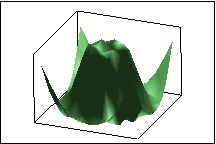### Parameters

You can specify values for the following parameters (in order):
• A (cannot equal 0)
• B (cannot equal 0)
• C (cannot equal 0)

## Egg carton (function 5)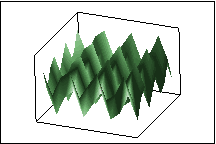### Parameters

You can specify values for the following parameters (in order):
• A (cannot equal 0)
• B (cannot equal 0)
• C (cannot equal 0)

## Hemisphere (function 6)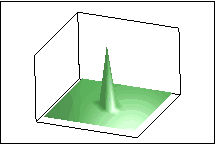### Equation

Where if z < 0, set z = 0

### Parameters

You can specify values for the following parameters (in order):
• A (cannot equal 0)
• B (cannot equal 0)
• C (cannot equal 0)
• R (cannot equal 0)

## Hill and dale (function 7)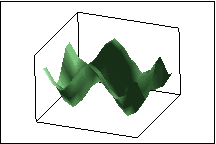### Parameters

You can specify values for the following parameters (in order):
• A (cannot equal 0)
• B (cannot equal 0)
• C (cannot equal 0)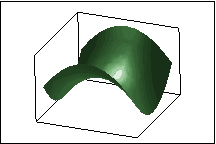### Parameters

You can specify values for the following parameters (in order):
• A (cannot equal 0)
• B (cannot equal 0)
• C (cannot equal 0)

## Wave (function 9)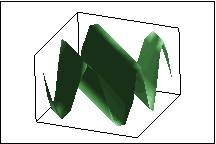### Parameters

You can specify values for the following parameters (in order):
• A (cannot equal 0)
• B (cannot equal 0)
• C (cannot equal 0)

## Custom functions

You can create your own custom functions by adding them to the bottom of USERFUNC.MAC, which is located with your Minitab program files in the Macros folder within your language folder. When you add a custom function to USERFUNC.MAC, you must assign your function a number between 10 and 1000. You will use this number to access the function in Calc > Make Mesh Data.

You can allow for optional parameters in the functions that you create, and set the values for these parameters in Calc > Make Mesh Data when you create the data. However, you should create reasonable default values for the parameters, in case parameter settings are not entered in Calc > Make Mesh Data. For more information on creating macros, go to Macros help.